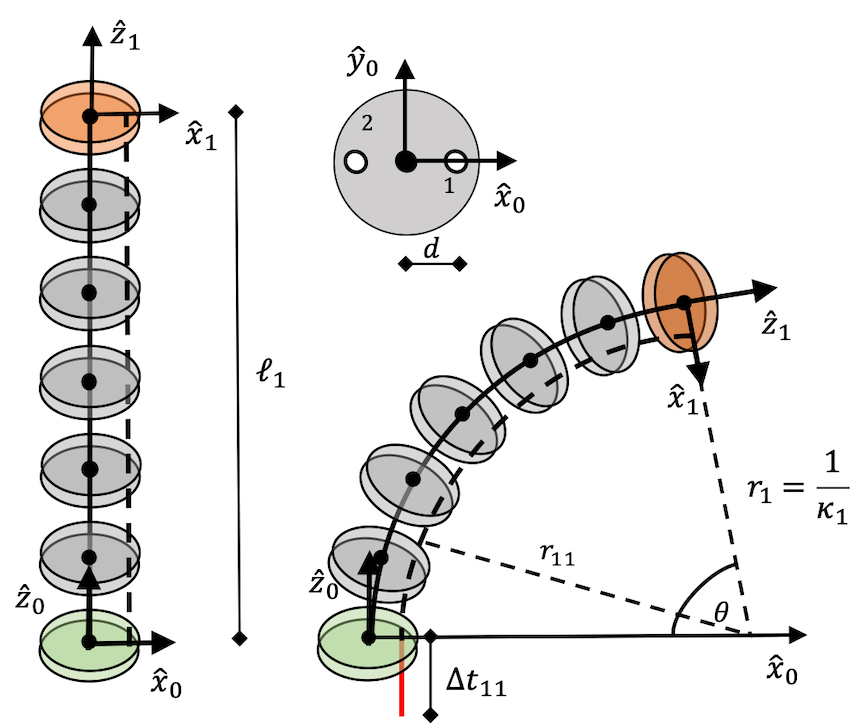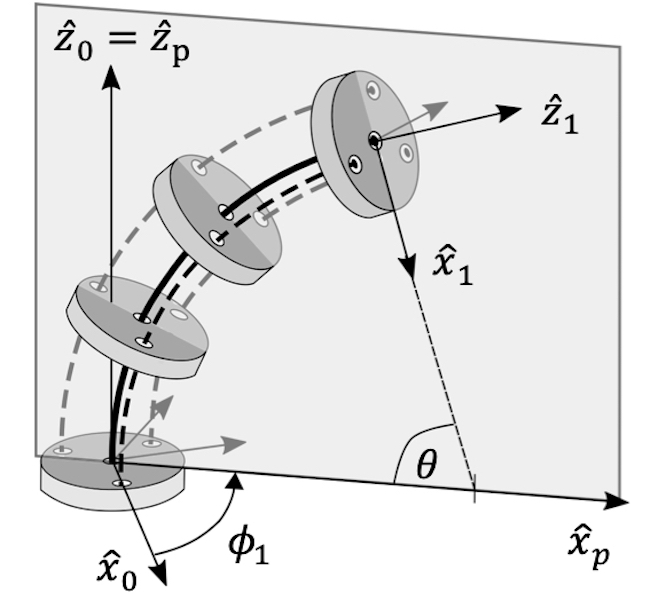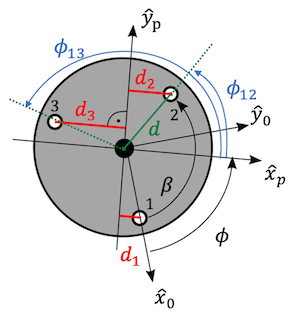# Constant-Curvature Model of Tendon-driven Continuum Robots

Today, we are looking at how tendon-driven continuum robots (TDCR) are modelled using the constant curvature kinematics framework introduced last week. In doing so, we assume that the TDCR undergoes a pure bending motion, i.e. has infinite torsional rigidity, and is not affected by gravitational effects. We also assume that the tendons follow a continuous curve, parallel to the backbone. Furthermore, we neglect friction in the tendon paths and any effects of external forces.

To determine the robot dependent mapping for a TDCR, we look at its $$m$$ segments. Each segment is actuated by a number of tendons $$N_{t}$$ terminating at a segment’s end disk.

We define the coordinate frame for each segment at the base disk positioned in its centre and choose the $$+ {\widehat{z}}_{0}$$-axis to point toward the end disk and tangent to the backbone. The $$+ {\widehat{x}}_{0}$$-axis is pointing toward the first tendon, such that pulling on the first tendon results in bending in the $${\widehat{x}}_{0}{\widehat{z}}_{0}$$-plane with positive curvature about the positive $${\widehat{y}}_{0}$$ axis. The tendons are numbered counterclockwise $$1\ldots N_{t}$$. The distance $$d$$ of the tendons to the centre of the disk is assumed to be constant per segment.

We further define the joint space of a TDCR as

$\mathbf{q} = \lbrack\Delta t_{11},\ldots,\Delta t_{1N_{t}},\ldots,\Delta t_{m1},\ldots,\Delta t_{mN_{t}}\rbrack$

where $$\Delta t_{ij}$$ is the tendon displacement of tendon $$j \in \lbrack 1,N_{t}\rbrack$$ in segment $$i \in \lbrack 1,m\rbrack$$. We define $$\Delta t_{ij} = \ell_{ij} - \ell_{i}$$ , where $$\ell_{i}$$ is the length of the i-th segment such that negative values of $$\Delta t_{ij}$$ indicate pulling on the tendon and positive values indicate releasing the tendon. Therefore, $$\ell_{ij}$$ represents the length of the $$j$$-th tendon enclosed by segment $$i$$. In its zero configuration, the TDCR is straight, i.e., the length of each tendon $$\ell_{ij} = \ell_{i}$$ and $$\mathbf{q} = \mathbf{0}$$.

## One Tendon Actuating a Segment

In the simplest case, a TDCR is composed of a single segment of fixed length $$\ell_{1}$$ and actuated by a single tendon as depicted here. Pulling on the tendon by $$\Delta t_{11}$$ causes the segment to bend into an arc with curvature $$\kappa_{1}$$. We seek in a relationship from joint space $$\mathbf{q} = \lbrack\Delta t_{11}\rbrack$$ to arc parameters $$\kappa_{1},\phi_{1},\ell_{1}$$. As we are only actuating with one tendon, the bending plane is fixed $$\phi_{1} = 0$$. As the segment has fixed length, $$\ell_{1}$$ is constant.For $$\Delta t_{11} \geq 0$$, the TDCR remains straight with $$\kappa_{1} = 0$$. To determine $$\kappa_{1}$$ for $$\Delta t_{ij} < 0$$, we look at the relationship between the tendon length $$\ell_{11}$$ and the backbone length $$\ell_{1}$$ which is constant. The tendon path is an arc with radius of curvature $$r_{11}$$, which is offset from the central backbone by distance $$d$$. We utilize the relationship between the arc described by the TDCR backbone and the tendon path as both share the central angle $$\theta$$.

$\begin{matrix} \theta = \frac{\ell_{1}}{r_{1}} \; \text{and} & \theta = \frac{\ell_{11}}{r_{1} - d} \Rightarrow \\ \ell_{11}r_{1} & = \ell_{1}(r_{1} - d) \Leftrightarrow \\ r_{1} & = \frac{\ell_{1}d}{\ell_{1} - \ell_{11}} \Leftrightarrow \\ \kappa_{1} & = \frac{\ell_{1} - \ell_{11}}{\ell_{1}d} = \frac{- \Delta t_{11}}{\ell_{1}d} \\ \end{matrix}$

## Planar Bending Segment

To achieve planar bending in two directions, two antagonistic tendons are used as depicted below. While the joint space is two-dimensional $$\mathbf{q} = \lbrack\Delta t_{11},\Delta t_{12}\rbrack$$, the tendon displacement is interdependent, i.e. $$\Delta t_{11} = - \Delta t_{12}$$. Pulling on one tendon requires releasing the other tendon in order to achieve bending. For $$\mathbf{q} = \lbrack 0,0\rbrack$$, $$\kappa_{1} = 0$$ and $$\phi_{1} = 0$$.The TDCR segment can either bend to the right $$\Delta t_{11} < 0$$, which implies $$\phi_{1} = 0$$, or to the left $$\Delta t_{12} < 0$$, which implies $$\phi_{1} = \pi$$. The curvature of the arc can be determined by utilizing the arcs described by the two tendon paths of length $$\ell_{11}$$ and $$\ell_{12}$$. Both of these arcs share the same central angle $$\theta$$ and are offset from the central backbone by $$d$$.

$\begin{matrix} \frac{\ell_{11}}{r_{1} - d} & = \frac{\ell_{12}}{r_{1} + d} \Leftrightarrow \\ \ell_{11}r_{1} + \ell_{11}d & = \ell_{12}r_{1} - \ell_{12}d \Leftrightarrow \\ r_{1} & = \frac{d(\ell_{11} + \ell_{12})}{\ell_{12} - \ell_{11}} \Leftrightarrow \\ \kappa_{1} & = \frac{\ell_{12} - \ell_{11}}{d(\ell_{11} + \ell_{12})} \\ \end{matrix}$

As the bending plane is determined by $$\phi_{1}$$, we require positive curvature and take the absolute value $$|\kappa_{1}|$$.

## Spatial Bending Segment

For a spatial bending segment, we look at a TDCR with minimum number of tendons, i.e., $$N_{t} = 3$$ as depicted below and derive the arc parameters for a single segment in the following. The joint space $$\mathbf{q} = \lbrack\Delta t_{11},\Delta t_{12},\Delta t_{13}\rbrack$$ is three-dimensional, but not all tendons can be actuated independently. In fact, to bend the segment, we can either pull on one tendon and release the other two by the same amount, or pull on two tendons and release the remaining one by the same amount. This dependency can be expressed by requiring $$\sum_{j}\Delta t_{1j} = 0$$ and consequently $$\ell_{1} = \frac{\sum_{j}^{}\ell_{1j}}{3}$$.For the robot dependent mapping $$\mathbf{q} \rightarrow \{\kappa_{1},\phi_{1},\ell_{1}\}$$, we assume that $$\ell_{1}$$ is constant, i.e. the segment has a fixed length and cannot extend or contract. The segment bends into the bending plane $${\widehat{x}}_{p}{\widehat{z}}_{p}$$ defined by $$\phi_{1}$$ about the $${\widehat{z}}_{0}$$-axis.To derive relationships for $$\kappa_{1}$$ and $$\phi_{1}$$, we establish relationships between the tendons and the central backbone within each disk first. The angular coordinate $$\phi_{1j}$$ of each tendon $$j$$ about $${\widehat{z}}_{0}$$ is related to $$\phi_{1}$$ according to the following equation

$\phi_{1j} = (j - 1)\beta - \phi_{1}$

where $$\beta = \frac{2\pi}{3}$$.

The projection of the $$j$$-th tendon on the segment bending plane is a curve with radius $$r_{1j}$$ (see dashed red line in figure below), which is offset by $$d_{j} = d\cos\phi_{1j}$$ from the central backbone. The radius of curvature of the central backbone $$r_{1}$$ is related to the radius of curvature of the j-th tendon according to

$$r_{1} = r_{1j} + d_{j}$$.We can use this relationship to determine the TDCR segment’s curvature by integrating the arc length $$s$$:

$\begin{matrix} \ell_{1j} & = \int ds_{ij} = \int(ds_{ij} - ds + ds) \\ & = \ell_{1} + \int_{\theta(0)}^{\theta(\ell_{1})}(r_{1j} - r_{1})d\theta \\ & = \ell_{1} - d_{j}(\theta(\ell_{1}) - \theta(0)) \\ \end{matrix}$

Rearranging and substituting the definition $$\Delta t_{1j}$$ yields the central angle of the arc $$\theta = \frac{- \Delta t_{1j}}{d_{j}}$$.

Using arc geometry $$\ell_{1} = r_{1}\theta$$, we can now determine the segment’s curvature as

$\kappa_{1} = \frac{- \Delta t_{1j}}{d_{j}\ell_{1}}$

Since the arc described by each tendon projection onto the bending plane share the same central angle $$\theta$$, we can establish kinematic compatibility conditions between two tendons, e.g., tendon $$1$$ and $$2$$:

$(\ell_{1} - \ell_{11})d_{2} = (\ell_{1} - \ell_{12})d_{1}$

Considering $$d_{1} = d\cos\phi_{1}$$ and $$d_{2} = d\cos(\beta - \phi_{1})$$ as well as trigonometric relationships, we can determine $$\phi_{1}$$ as

$$\phi_{1} = \text{atan2}(\Delta t_{11}\cos\beta - \Delta t_{12}, - \Delta t_{11}\sin\beta)$$.

Equivalently, we can express the kinematic compatibility condition between tendon $$1$$ and $$3$$ or tendon $$2$$ and $$3$$ and determine $$\phi_{1}$$. Which combination to choose depends on $$\mathbf{q}$$. Note, that we use the two-argument arctangent $$\text{atan2}(y,x) \in \lbrack 0,2\pi\rbrack$$ as the single-argument $$\text{atan}(x) \in \lbrack 0,\pi\rbrack$$ cannot distinguish all quadrants.

## Extensible Spatial Bending Segment

For TDCR segments that can spatially bend and extend, the joint space becomes $$\mathbf{q} = \lbrack\Delta t_{11},\cdots,\Delta t_{1N_{t}},\Delta\ell_{1},\cdots,\Delta t_{m1},\cdots,\Delta t_{mN_{t}},\Delta\ell_{m}\rbrack$$, where $$\Delta\ell_{i}$$ describes the relative change in length of the central backbone w.r.t. the backbone length in the robot’s zero position $$\ell_{i\{ 0\}}$$. Consequently, the length of each segment can be determined as $$\ell_{i} = \ell_{i\{ 0\}} + \Delta\ell_{i}$$. The robot independent mapping then follows the approach described before.

## Multi-segment TDCR

While we looked at the establishment of the robot independent mapping for single segment TDCR in this section, note that the approach for multi-segment TDCR is equivalent for each of the $$m$$ segments. In the constant curvature kinematics framework, we assume that the tendons only actuate the segment they terminate at. In other words, we assume that there is no effect of tendon coupling and each segment bends independently. Therefore, each segment can be individually looked at to determine the arc parameter triplet.

## Remarks

The constant curvature approximation comes at a cost for TDCR. The kinematic formulation cannot account for the elastic properties of the backbone. It cannot account for any external forces or moments or gravity and is therefore limited in its application.

While the configuration space representation theoretically requires an inﬁnite number of parameters due to the continuous nature of their backbones, a reduced set of parameters can be chosen to represent the robot. Furthermore, different assumptions of increasing complexity regarding the kinematics and statics of the robot’s backbone and tendons can be included in the mapping between joint and configuration space. As a result, existing kinematic and static models are often tailored to a specific TDCR design and make trade-oﬀs between accuracy and computation speed depending on the envisioned application.

Further Reading: You want to dig deeper into constant curvature modeling of TDCR? Webster & Jones1 published a beginner-friendly review on modeling continuum robots using the constant curvature framework. Simaan et al.2 describe the kinematics for multi-segment TDCR using push-pull rods instead of tendons. With Rao3, Peyron, and Lilge, we published a survey on modeling of TDCR going into depth of different techniques.

1. Webster, R.J. & Jones, B.A., 2010. Design and Kinematic Modeling of Constant Curvature Continuum Robots: A Review. The International Journal of Robotics Research, 29(13):1661–1683. doi: 10.1177/0278364910368147

2. Simaan, Nabil, Kai Xu, Ankur Kapoor, Wei Wei, Peter Kazanzides, Paul Flint, and Russell Taylor. 2009. Design and Integration of a Telerobotic System for Minimally Invasive Surgery of the Throat. The International Journal of Robotics Research 28 (9):1134–53. doi: 10.1177/0278364908104278

3. P. Rao, Q. Peyron, S. Lilge, and J. Burgner-Kahrs, 2021. How to Model Tendon-Driven Continuum Robots and Benchmark Modelling Performance. Frontiers in Robotics & AI, 7:1-20. doi: 10.3389/frobt.2020.630245